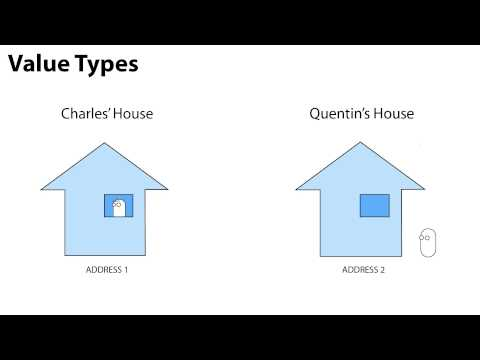# Data Types

#### 난이도: 초급

Learn the important differences between Value and Reference data types, in order to better understand how variables work.## Data Types

초급 Scripting

### Code snippet

``````using UnityEngine;
using System.Collections;

public class DatatypeScript : MonoBehaviour
{
void Start ()
{
//Value type variable
Vector3 pos = transform.position;
pos = new Vector3(0, 2, 0);

//Reference type variable
Transform tran = transform;
tran.position = new Vector3(0, 2, 0);
}
}``````
``````#pragma strict

function Start ()
{
//Value type variable
var pos : Vector3 = transform.position;
pos = new Vector3(0, 2, 0);

//Reference type variable
var tran : Transform = transform;
tran.position = new Vector3(0, 2, 0);
}``````
``````import UnityEngine
import System.Collections

public class DatatypeScript(MonoBehaviour):

private def Start():
//Value type variable
pos as Vector3 = transform.position
pos = Vector3(0, 2, 0)

//Reference type variable
tran as Transform = transform
tran.position = Vector3(0, 2, 0)``````

#### 관련 자습서